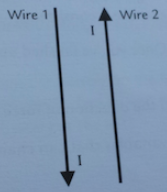# Problem: Two current-carrying wires are exactly parallel to one another and both carry 3.0 A of current. The two wires are separated by a distance of 25 cm. The current in wire 1 moves down while the current in wire 2 moves up. What is the magnitude of the magnetic force per unit length caused by wire 1 on wire 2? What is the direction of the magnetic force caused by wire 1 on wire 2?

###### FREE Expert Solution

Force per unit length,

$\overline{)\begin{array}{rcl}\frac{\mathbf{F}}{\mathbf{∆}\mathbf{L}}& \mathbf{=}& \frac{{\mathbf{\mu }}_{\mathbf{0}}{\mathbf{I}}_{\mathbf{1}}{\mathbf{I}}_{\mathbf{2}}}{\mathbf{2}\mathbf{\pi r}}\end{array}}$

I1 = I2 = 3.0 A

r = 25 cm (1 m / 100 cm) = 0.25 m

μ0  = 4π × 10-7 T•m/A

82% (215 ratings)###### Problem Details

Two current-carrying wires are exactly parallel to one another and both carry 3.0 A of current. The two wires are separated by a distance of 25 cm. The current in wire 1 moves down while the current in wire 2 moves up. What is the magnitude of the magnetic force per unit length caused by wire 1 on wire 2? What is the direction of the magnetic force caused by wire 1 on wire 2?